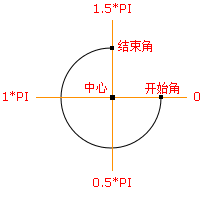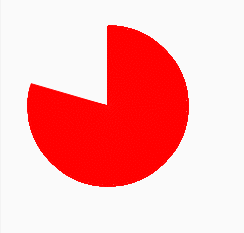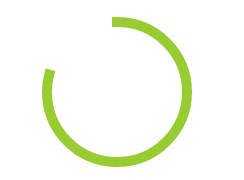# canvas绘制旋转的圆环百分比进度条

### 设置圆环的颜色和大小

``````ctx.beginPath();
ctx.strokeStyle = '#99CC33';
ctx.lineCap = 'square';
ctx.closePath();
ctx.fill();
ctx.lineWidth = 10.0;``````

### 设置圆环百分比进度从上面这个图，我们可以看出canvas的开始角并不在我们印象中圆的顶点位置，所以我们要设置开始角的位置在1.5*Math.PI开始，可以是顺时针，也可以是反时针方向。考虑到现在市面上的圆环进度条都是以顺时针开始。
`context.arc(x,y,r,sAngle,eAngle,counterclockwise);`

`<canvas id="bg" width="200" height="200"></canvas>`

### 第一种：圆饼百分比代码如下：
```var bg = document.getElementById('bg');
var cxt = bg.getContext('2d'); //创建context对象
cxt.fillStyle = '#FF0000'; //设置或返回用于填充绘画的颜色、渐变或模式 染成红色
cxt.strokeStyle="#ff0000"; //设置或返回用于笔触的颜色、渐变或模式
//cxt.lineCap = 'square'; //设置或返回线条的结束端点样式
cxt.beginPath(); //起始一条路径，或重置当前路径
cxt.moveTo(100,100); //把路径移动到画布中的指定点，不创建线条

function draw(current){

//cxt.lineTo(100,0); //添加一个新点，然后在画布中创建从该点到最后指定点的线条
cxt.arc(100,100,80,-0.5*Math.PI,current*(2*Math.PI) - Math.PI/2,false); //绘制一个中心点为（100,100），半径为100，起始点为1.5*Math.PI,终点为2*Math.PI,顺时针的1/4圆
cxt.closePath(); //创建从当前点回到起始点的路径
cxt.fill();//填充当前绘图（路径）
//cxt.lineWidth = 10.0; //设置或返回当前的线条宽度
cxt.stroke(); //绘制已定义的路径
}
//draw(0.6);
var t=0;
var timer=null;
timer = setInterval(function(){
if(t>now){
draw(now);//最后一次绘制
clearInterval(timer);
}else{
draw(t);
t+=0.005;
}
},5);
}
timer=null;```

### 第二种：圆环百分比代码如下：
```var bg = document.getElementById('bg');
var ctx = bg.getContext('2d');
var circ = Math.PI * 2;
var quart = Math.PI / 2;
var imd = null;
var circ = Math.PI * 2;
var quart = Math.PI / 2;

ctx.beginPath();
ctx.strokeStyle = '#99CC33';
ctx.lineCap = 'square';
ctx.closePath();
ctx.fill();
ctx.lineWidth = 10.0;

imd = ctx.getImageData(0, 0, 240, 240);
function draw(current){
ctx.putImageData(imd, 0, 0);
ctx.beginPath();
ctx.arc(120, 120, 70, -(quart), ((circ) * current) - quart, false);
ctx.stroke();
}
//draw(0.6);
var t=0;
var timer=null;